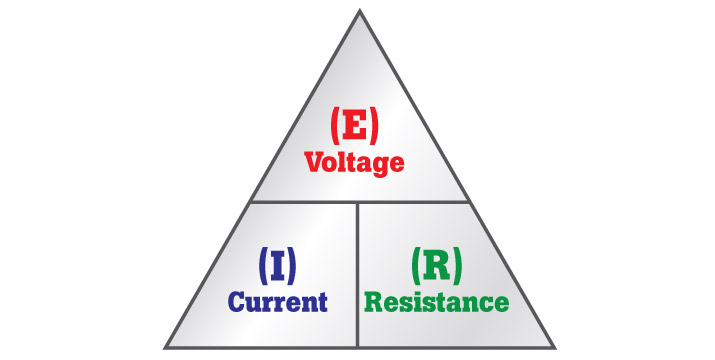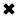# Ohm's Law - Voltage, Current, Resistance#### Ohm’s law formulas are used around the world to determine electromotive force.

Ohm’s law is the fundamental equation that explains the relationship between voltage, current and resistance in ac circuits. The power dissipated in a load resistance(R) is defined as the product of the electric current and applied voltage (P=VI). By substitution other relationships of power can be derived by applying ohms law.

Before describing ohm’s law, let’s define the three main parameters that are fundamental to any electric circuit.

Voltage: Also known as a Electric Potential Difference, is a driving force in any circuit; it is measured in Volts (V).

Current: Is the flow of electrons from a higher potential to a lower potential, its unit is Ampere (A)

There must be a closed loop or path for the existence of current flow

Resistance: Is the characteristic of a medium that opposes the flow of current. The unit of resistance is

Ohms & it is represented by the Greek letter Ω (Omega).

Applications of Ohm’s Law
Ohm’s law helps us in determining either proportional to the voltage, current or impedance or resistance of a linear electric circuit when the other two quantities are known to us. It also makes power calculation simpler.

Georg Ohm

Georg Simon Ohm, the inventor of Ohm's Law, was born in Erlangen, Bavaria, on March 16, 1787. His Father, a successful mechanic, wanted him to study science however Ohm became a high school teacher. Ohm had aspirations to join university, but at that time the only way to join university was providing important invention through scientific research. Since the science of electricity was at the infancy stage ohm decided to study the behavior of current in resistive circuits was like water flow. Since equipment was technically unaffordable for ohm, he made much of his own. Ohm determined experimentally that the amount of electricity in terms of current that flows along a wire in an electrical device was directly proportional to its cross sectional area and inversely proportional to its length from this results ohms was able to define resistance and show that there is a simple relationship proportioanl to the voltage, resistance and current.; this result now known as the ohms law; the most fundamental relationship in circuit theory. However when published in 1827 ohms results were met with ridicule as a result not only did ohm miss out on a university appointment he was also forced to resign from his high school teaching position. While ohm was living in a poverty and shame, his work on dc circuits became known and appreciated outside Germany .In 1852; Ohm was appointed a member of the Royal Society. Finally, in 1849, he was appointed as a professor at the University of Munich, where he was at last recognized for his important contributions.According to Ohm, he determined experimentally that potential energy current in resistive circuit points is directly proportional to its applied voltage and inversely proportional to its resistance. This is what is known as Ohm's Law.

In equation form, Ohm’s law states the current (I) that flows through the Resistor(R) is:

I=E/R   [amps, A]

Where   E is applied voltage in volts

R is the resistance in ohms

I is the current in amperes

From the above formula for we can see that as the applied voltage increases the current also increases, as the resistance increases the current decreases.Fig. 1aFig. 1bFig. 2

To conduct this Ohm's Law experiment:

1. First, connect a battery/source in series with an Ammeter and a fixed value Resistor
2. Then, connect a voltmeter in parallel as shown in Fig. 2. Then, Record the current, voltage and resistance values.
3. Then, disconnect the batter and replace the resistor with a higher value one. Record the values of current for different value of Resistors.
4. Finally, Observe how the current varies for different values of resistors.

For a fixed resistance doubling the voltage doubles the current measured, while tripling the voltage triples the current. For a fixed voltage, doubling the resistance halves the current, while tripling the resistance reduces the current to 1/3 of its original value and so on.

What to validate using Ohm’s Law

Ohm’s Law can be used to validate the static values of electrical and electronic circuit components, current levels, voltage supplies, and voltage drops. If, for example, a test instrument detects a higher than normal current measurement, it could mean that resistance has decreased or that voltage has increased, causing a high-voltage situation. This could indicate a supply or circuit issue.

In direct current (dc) circuits, a lower than normal current measurement could mean that the voltage has decreased, or circuit resistance has increased. Possible causes for increased resistance are poor or loose connections, corrosion and/or damaged components.

With Ohm’s Law, loads within a circuit draw on electrical current. Loads can be any sort of component: small electrical devices, computers, household appliances or a large motor. Most of these components (loads) have a nameplate or informational sticker attached. These nameplates provide safety certification and multiple reference numbers.

Technicians refer to nameplates on components to learn standard voltage and current values. During testing, if technicians find that customary values do not register on their digital multimeters or clamp meters, they can use Ohm's Law to detect what part of a circuit is faltering and from that determine where a problem may lie.

The basic science of circuits

Circuits, like all matter, are made of atoms. Atoms consist of subatomic particles:

• Protons (with a positive electrical charge)
• Neutrons (no charge)
• Electrons (negatively charged)

Atoms remain bound together by forces of attraction between an atom's nucleus and electrons in its outer shell. When influenced by voltage, atoms in a circuit begin to reform and their components exert a potential of attraction known as a potential difference. Mutually attracted loose electrons move toward protons, creating a flow of electrons (current). Any material in the circuit that restricts this flow is considered resistance.

##### Basic Electricity Handbook, Vol. 1• FULLY ILLUSTRATED
• GREAT FOR SCHOOL PROJECTS
• GREAT PRICE: \$5.99
• ...

This 100+ page e-book is a great guide for those who have a basic interest in the field of electricity. This well-illustrated e-book, coupled with some basic knowledge of electricity, will give you a broad theoretical background in this fundamental subject.

CONTENTS
• FUNDAMENTALS OF ELECTRICITY
• HOW DOES ELECTRICITY WORK?
• HOW IS ELECTRICITY GENERATED?
• WHERE DOES ELECTRICITY COME FROM?
• BASIC HOME WIRING
• ELECTRICITY FOR STUDENTS
• ENERGY SAVING TIPS

##### TRAINING EF COURSES

Content Community Connection
Top